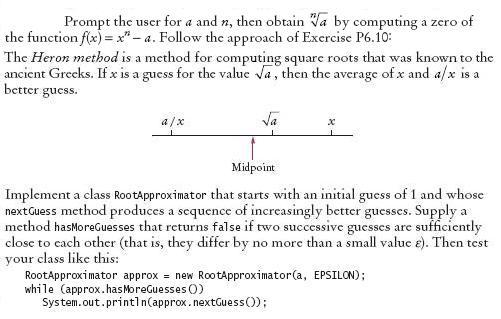The best known iterative method for computing the roots of a function f (that is, the x-values for which f(x) is 0) is Newton-Raphson approximation. To find the zero of a function whose derivative is also known, compute

xnew = xold - f(xold)/f'(xold)

For this exercise, write a program to compute nth roots of floating-point numbers.Complete the following files:

### RootCalculator.java

Use the following file:

RootApproximatorTester.java

```public class RootApproximatorTester
{
public static void main(String[] args)
{
double a = 10000;
final double EPSILON = 1000;
RootApproximator approx = new RootApproximator(a, 4, EPSILON);
System.out.println(approx.nextGuess());
System.out.println("Expected: 1");
System.out.println(approx.nextGuess());
System.out.println("Expected: 2500.75");
System.out.println(approx.hasMoreGuesses());
System.out.println("Expected: true");
approx.nextGuess();
System.out.println(approx.hasMoreGuesses());
System.out.println("Expected: false");
}
}
```